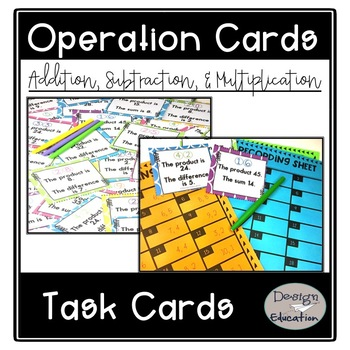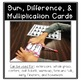Subject
Resource Type
Format
PDF (14 MB|22 pages)
Standards
\$2.00
• Product Description
• Standards

Looking for a way to encourage a deep understanding of number sense? This Sum, Difference, and Product task card set promotes critical thinking and understanding of how numbers work together. Each card requires the student to determine what 2 numbers the statements on the card. Students will master mental addition, subtraction, and multiplication in no time using these problem solving cards!

What's Included:

• 42 Color Sum, Difference, and Multiplication Cards
• 42 Black and White Sum, Difference, and Multiplication Cards (to meet your printing needs)
• Student Recording Sheet
• Teacher Suggestions

Many Options for Use:

• Extensions
• Whole group
• Exit tickets
• Small group
• Math Center
• Morning Work
• Homework
• Early Finishers

You Will Love My Other Math Products:

Math Stem Tub

Counting Coins Dominoes

to see state-specific standards (only available in the US).
Add and subtract within 20, demonstrating fluency for addition and subtraction within 10. Use strategies such as counting on; making ten (e.g., 8 + 6 = 8 + 2 + 4 = 10 + 4 = 14); decomposing a number leading to a ten (e.g., 13 - 4 = 13 - 3 - 1 = 10 - 1 = 9); using the relationship between addition and subtraction (e.g., knowing that 8 + 4 = 12, one knows 12 - 8 = 4); and creating equivalent but easier or known sums (e.g., adding 6 + 7 by creating the known equivalent 6 + 6 + 1 = 12 + 1 = 13).
Multiply one-digit whole numbers by multiples of 10 in the range 10–90 (e.g., 9 × 80, 5 × 60) using strategies based on place value and properties of operations.
Fluently add and subtract within 1000 using strategies and algorithms based on place value, properties of operations, and/or the relationship between addition and subtraction.
Explain why addition and subtraction strategies work, using place value and the properties of operations.
Fluently add and subtract within 100 using strategies based on place value, properties of operations, and/or the relationship between addition and subtraction.
Total Pages
22 pages
Included
Teaching Duration
N/A
Report this Resource to TpT
Reported resources will be reviewed by our team. Report this resource to let us know if this resource violates TpT’s content guidelines.
• Ratings & Reviews
• Q & A

Teachers Pay Teachers is an online marketplace where teachers buy and sell original educational materials.